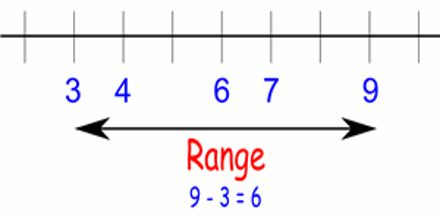Mathematic

# Lecture on RangeGeneral objective of this lecture is to present on Range. Range – the difference between the greatest and the least value in a set of numbers. The difference between the lowest and highest values. In {4, 6, 9, 3, 7} the lowest value is 3, and the highest is 9, so the range is 9 − 3 = 6. Range can also mean all the output values of a function. This lecture briefly describe on how to Find the Range in a Group of Numbers.